International Journal of Economics, Finance and Management Sciences
Volume 5, Issue 1, February 2017, Pages: 15-23

Interest Rate Risk Management of Commercial Banks in Bangladesh Based on IS (Interest Sensitivity) GAP Analysis

Raad Mozib Lalon, Md. Bazlul Kabir

Department of Banking & Insurance, University of Dhaka, Dhaka, Bangladesh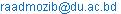(R. M. Lalon)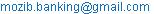(Md. B. Kabir)

Raad Mozib Lalon, Md. Bazlul Kabir. Interest Rate Risk Management of Commercial Banks in Bangladesh Based on IS (Interest Sensitivity) GAP Analysis. International Journal of Economics, Finance and Management Sciences. Vol. 5, No. 1, 2017, pp. 15-23. doi: 10.11648/j.ijefm.20170501.12

Received: October 20, 2016; Accepted: November 2, 2016; Published: December 23, 2016

Abstract: This paper on the interest rate risk management of a bank will provide a detailed picture of risk management of bank because it is one of the concerned factors for every bank. This study will also indicate any shortfall of bank in terms of interest rate risk management and offer suitable recommendations. Interest rate risk is the exposure of a bank's financial condition to adverse movements in interest rates. Accepting this risk is a normal part of banking and can be an important source of profitability and shareholder value. However, excessive interest rate risk can pose a significant threat to a bank's earnings and capital base. Changes in interest rates affect a bank's earnings by changing its net interest income and the level of other interest sensitive income and operating expenses. Changes in interest rates also affect the underlying value of the bank's assets, liabilities, and off-balance-sheet (OBS) instruments because the present value of future cash flows (and in some cases, the cash flows themselves) change when interest rates change. Accordingly, an effective risk management process that maintains interest rate risk within prudent levels is essential to the safety and soundness of banks.

Keywords: Irr, Nim, Nii, Is Gap, Car, Crm, Mcr, Rwa

Contents

1. Introduction

Interest rate risk (IRR) is defined as the change in a bank’s portfolio value due to interest rate fluctuations. It is the potential loss from unexpected changes in interest rates, which can significantly affect a bank’s profitability and market value of equity. Interest rate risk in banking is originated by a mismatching of assets and liabilities maturities and interest rate re-pricing on assets and liabilities. When the interest rate changes in the financial marketplace, the sources of revenue and their expenses must also change. If interest rates rise, the cost of funds increases more rapidly than the yield on assets. It reduces net income. So interest rate fluctuation may have a negative impact on the economic and financial statement through assets, liabilities, and off-balance sheets positions related to interest rates. If the exposure is not managed properly it can reduce both the profitability and shareholder value.

2. Objectives of the Study

The objective of this paper is:

To find out the interest rate risk of selected commercial banks and risk management techniques adopted by those banks.

To know about the way how the bank progress in case of interest rate risk

To know about the position of ABL (Agrani Bank Ltd) and PBL (Prime Bank Ltd) in interest rate GAP

To know about the Net Interest Margin of ABL and PBL

To analyze which factors the bank consider to minimize the risk

To examine the last 8 years data to overlook its risk position

To find out the relation among various factors which affect the risk

To find out the sensitivity and mismatched maturity of the bank assets and liabilities.

3. Methodology

3.1. Research Type

This is a descriptive research which is relevant to an inquisitive study as it require some analysis on the performance of different banks in terms of interest rate risk management.

3.2. Types of Data

For conducting this research I will only utilize the secondary sources of data as shown below:

Various research, books, articles on Interest risk guidelines & management

Website of Agrani bank and Prime bank ltd

Annual report of Agrani bank and Prime bank ltd

Policy of interest rate risk management of Agrani bank and Prime bank ltd

3.3. Data Analysis Tools

In order to keep the analysis precise and point to point, I have used following tools to analyze the data.

3.3.1. Ratio Analysis

NIM(Net Interest Margin)

Interest sensitive asset to liabilities

3.3.2. Gap Analysis

Both Interest Sensitive Gap & Relative IS Gap have beenconducted.

4. Measurement of Interest Rate Risk

Managing interest rate risk requires a clear understanding of the amount at risk and the impact of changes in interest rates on this risk position. To make these determinations, sufficient information must be available to permit appropriate action to be taken within acceptable, often very short, time periods. The longer it takes an institution to eliminate or reverse an unwanted exposure, the greater the possibility of loss. Each institution needs to use risk measurement techniques that accurately and frequently measure the impact of potential interest rate changes on the institution. In choosing appropriate rate scenarios to measure the effect of rate changes, the institution should consider the potential volatility of rates and the time period within which the institution could practically react to close the position. Interest rate risk measurement techniques are: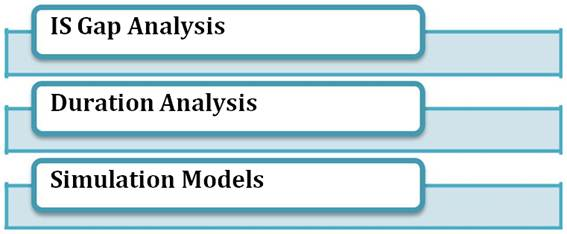Source: Author’s own design

Figure 1. Interest rate risk measurement techniques.

Each institution should use at least one, and if possible a combination of these techniques in managing its interest rate risk exposure. Each technique provides a different perspective on interest rate risk, has distinct strengths and weaknesses, and is more effective when used in combination with another. These techniques are discussed below:

4.1. Interest Sensitive (IS) Gap Management

The interest sensitivity gap was one of the first techniques used in asset liability management to manage interest rate risk. The interest rate sensitivity gap classifies all assets, liabilities and off balance sheet transactions by effective maturity from an interest rate reset perspective. The interest rate sensitivity gap compares the amount of assets and liabilities in each time period in the interest rate sensitivity gap table. This comparison gives an approximate view of the interest rate risk of the balance sheet being analyzed. If management feels its institution is excessively exposed to interest rate risk, it will try to match as closely as possible the volume of assets that can be repriced as interest rate change with the volume of deposits and other liabilities whose rates can also be adjusted with market conditions during the same time period.

Table 1. Risk and response of a bank for interest- sensitive gap.

 Types of IS gap Risk Possible Management Response Asset Sensitive if Interest Sensitive Assets > Interest Sensitive Liabilities Losses if interest rates fall because the bank´s net interest margin will be reduced. i.   Do nothing (perhaps interest rates will rise or be stable). ii.  Extend asset maturities or shorten liability maturities. iii. Increase interest-sensitive liabilities or reduce interest-sensitive assets Liability Sensitive if Interest Sensitive Asset < Interest Sensitive Liabilities Losses if interest rates fall because the bank´s net interest margin will be reduced. i.   Do nothing (perhaps interest rates will rise or be stable). ii.  Extend asset maturities or shorten liability maturities. iii. Increase interest-sensitive liabilities or reduce interest-sensitive assets.

In dealing with interest rate risk, one important goal is to insulate profits-net income after taxes and all other expenses-from the damaging effects of fluctuating interest rates. No matter which way interest rates go, managers of financial institutions want profits that achieve the level desired and are stable. To accomplish this particular goal, management must concentrate on those elements of the institution's portfolio of assets and liabilities that are most sensitive to interest rate movements. In order to protect profits against adverse interest rate changes, then, management seeks to hold fixed the financial firm's Net Interest Margin (NIM).

4.2. Calculation of Net Interest Margin

The net Interest margin can be expressed as a performance metric that examines the success of a firm’s investment decisions as contrasted to its debt situations. A negative Net Interest Margin indicates that the firm was unable to make an optimal decision, as interest expenses were higher than the amount of returns produced by investments. The Net Interest Margin is calculated as:

Net Interest Margin = Net Interest Income ÷ Total Earning Assets

Where,

Net Interest Income = Total interest income - Total interest expense

Total earning asset

2.   Balance with other Banks and Financial institutions

3.   Money at Call and short notice

4.   Investmen

Table 2. Calculation of Net Interest Margin.

 (BDT in million) Year Net InterestIncome Total Earning Asset Net Interest Margin 2008 3857.5 159782.91 2.41% 2009 4038.8 168567.30 2.40% 2010 6910.9 212760.81 3.25% 2011 1046.87 287014.80 3.65% 2012 3982.73 313076.52 1.27% 2013 1265.25 367486.15 0.34% 2014 692.52 407771.36 0.17% 2015 1185.9 473014.84 0.25%

Source: Agrani Bank´s annual report.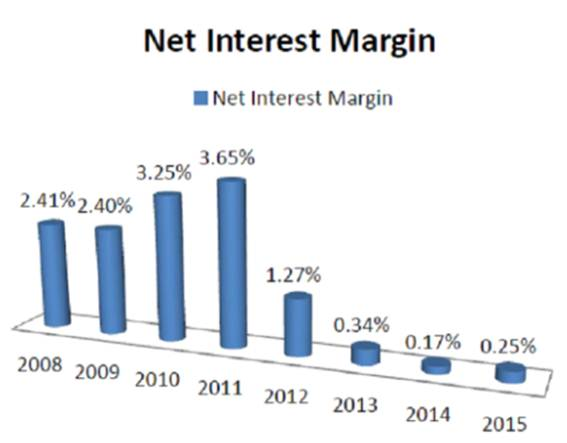Source: Annual report of Agrani Bank Ltd.

Figure 2. Net Interest Margin of Agrani Bank Ltd.

From the figure we can see that the Net Interest Margin was periodically increased from the year 2009-2011 which indicate that ABL was successful enough to make its investment decisions compared to its debt situations. But from the year 2012-2015 we can see that the Net Interest Margin has fallen significantly compared to previous years which indicates that ABL failed to make an optimal decision which means its interest expense was large enough compared to its interest income. We know that interest income fluctuate each year with the amount of cash a company keeps on hand and the general level of interest rates as set by the central bank. So we can assume or take the decision that in those years, Agrani Bank Ltd. keeps a large amount of cash on hand instead of investing it. For this reason the net interest margin falls in those years.

4.3. Interest Sensitivity Analysis (GAP Management) of ABL

For getting an overview of the calculation of interest sensitivity of a bank here I only do the calculation of interest sensitivity of a bank for 2015 and for rest of the years I only show the total interest sensitivity position of the bank by a chart. Here I actually try to show the interest sensitivity position of a bank by periodic basis and try to show the position of net worth due to change of increase or decrease of interest rate change.

From above table we can see that ABL has total interest sensitive assets of BDT 473014.6 million and total interest sensitive liabilities of BDT 520675.57 million Tk. As interest-sensitive liabilities are larger than asset by BDT 47660.97 million the bank is liability sensitive. So, the bank's net interest margin should reduce if interest rates rise as liability increase by more than the resulting increase in asset revenues. On the other hand, if interest rates fall, the bank's net interest margin will rise as asset revenues increases faster than liability costs.

If market interest rates suddenly increase by 1% point. Then the Bank will suffer a net interest income loss of approximately -

Change in Net Interest Income = Change in Interest RateSize of Cumulative Gap

=(.01)(-47660.76 million)

=- 476.61 million

So ABL has a positive gap in the nearest period and therefore would benefit if interest rates rise. In the next period it has a slightly negative gap and would therefore benefit of interest rate fall. However, its cumulative gap is still negative. The third period is positive gap and hence the bank would benefit if interest rates rises. In the final period the gap is negative and the bank would benefit if interest rates fall. Its cumulative gap is also negative and also shows that falling Interest rates would be beneficial to the bank overall.

Now here I have shown 8 years data to calculate the interest sensitive gap position of ABL. The results are shown on the following table:

Table 3. Interest Sensitivity Analysis on maturity basis.

 (BDT in million) Particulars Up to 1month Over 1 to3 months Over 3 to12 months Over 1 year to 5 years Over 5 years Total Assets: Balance withother banks &FIS 2937.59 8500 5315.71 4170 _ 20923.06 Money at call 1592.23 _ _ _ _ 1592.23 Investment 15.97 83502.53 33668.24 41446.49 47064.24 205697.47 Loans &Advances 29731.33 31271.45 78120.06 39895.93 65783.07 244801.84 Total asset 34277.12 123273.98 117103.98 85512.42 112847.31 473014.6 Liabilities: Borrowings 7753.37 _ 1074.23 0.57 8828.17 Deposits 48450.74 103938.52 92279.81 165600.40 29706 439975.47 Other liabilities 566.19 817.09 6616.74 34165.90 29706.01 71871.93 Total liability 56770.30 104755.61 98896.55 200840.53 59412.58 520675.57 Interest sensitivegap -22493.18 18518.37 18207.43 -115328.11 53434.73 Cumulative gap -22493.18 -3974.81 14232.62 -101095.49 -47660.76

Source: Agrani Bank´s annual report.

Table 4. Interest Sensitive gap.

 (BDT in million) Year Interest Sensitive Assets Interest Sensitive Liabilities Interest Sensitive Gap Situation Risk 2008 159782.91 182328.30 -22545.39 Liability Sensitive Losses if interest rate rise 2009 168567.30 202621.94 -34054.64 Liability Sensitive Losses if interest rate rise 2010 212760.81 249134.74 -36373.93 Liability Sensitive Losses if interest rate rise 2011 287014.80 322878.08 -35863.28 Liability Sensitive Losses if interest rate rise 2012 313076.52 371552.94 -58476.42 Liability Sensitive Losses if interest rate rise 2013 367486.15 408515.65 -41029.51 Liability Sensitive Losses if interest rate rise 2014 407771.31 455304.22 -47532.91 Liability Sensitive Losses if interest rate rise 2015 473014.60 520675.57 -47660.97 Liability Sensitive Losses if interest rate rise

Source: Agrani Bank´s annual report.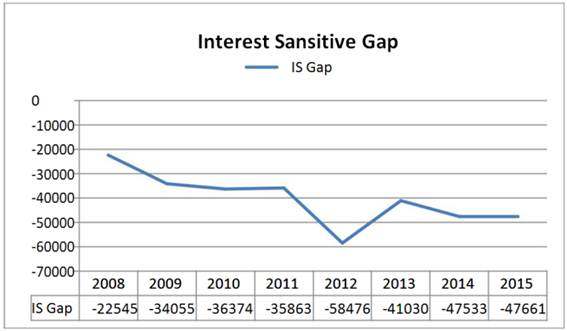Source: Agrani Bank´s annual report

Figure 3. Interest Sensitive GAP.

In the above figure all the values are negative that means from the year 2008-2015 ABL has a negative gap which indicate ABL's Interest sensitive liabilities are more than Interest sensitive assets. So we can take the decision that it will be benefited if the bank lowers the interest rates. These results are found considering overall maturity of the interest sensitive assets and liabilities. But when considering the different maturity period it may benefit by both lowering and rising the interest rates. In the figure we can see that from the year 2008 to 2015 the bank is almost in a continuous decreasing position but in the year 2012 gap was significantly decreased which indicates that most probably in that time bank would fail to make proper investment decision or to reprice the assets and liabilities.

4.3.1. Interest Sensitivity Ratio Analysis

An interest sensitivity ratio of less than 1 tells us that the institution is a liability sensitive institution, while an interest sensitivity ratio greater than unity points to an asset sensitive institution. To find out the interest sensitive position of the Bank we can calculate both the Relative IS Gap and the Interest Sensitivity Ratio.

i. Relative IS Gap

The relative interest-sensitive gap is the interest-sensitive gap divided by the bank size or total assets. In case of positive interest-sensitive gap, a relative IS Gap is also positive. On the other hand, in case of a negative interest-sensitive gap, a relative IS Gap is also negative.

Relative IS Gap = Is Gap ÷ Total Asset

To know the Relative IS Gap position of the bank I have analyzed 8 years data. The results are given below:

Table 5. Relative IS Gap.

 (BDT in million) Year IS Gap Total Assets Relative IS Gap 2008 -22545.39 187320.00 -0.12 2009 -34054.64 214062.87 -0.16 2010 -36373.93 264852.03 -0.14 2011 -35863.28 348820.71 -0.10 2012 -58476.42 378716.42 -0.15 2013 -41029.51 444156.58 -0.09 2014 -47532.91 494870.83 -0.10 2015 -47660.97 565351.46 -0.08

Source: Agrani Bank´s annual report.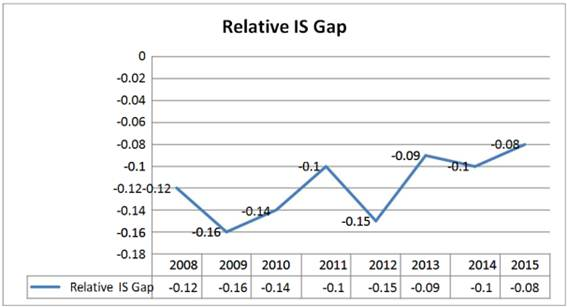Source: Agrani Bank´s annual report.

Figure 4. Relative IS GAP.

A Relative IS Gap greater than zero means the institution is asset sensitive while a negative Relative IS Gap describes a liability sensitive financial firm. From the above table we can see that the Relative IS Gap is negative and less than zero. So we can take the decision that Agrani Bank Ltd. is a liability sensitive institution. we can also see that the relative IS Gap is very high in the year 2012 and 2009 which is the result of large interest sensitive gap in those years which is described earlier.

ii. Interest Sensitivity Ratio

The interest-sensitivity ratio is just the ratio of interest-sensitive assets to interest sensitive liabilities. In case of positive interest-sensitive gap, an interest sensitivity ratio is greater than one. On the other hand, in case of a negative interest-sensitive gap, an interest sensitivity ratio less than one

Interest Sensitivity Ratio = Interest Sensitive Assets ÷ Interest Sensitive Liabilities

8 years Interest Sensitivity Ratio of Agrani Bank Ltd. is given below.

In this instance an Interest Sensitivity Ratio less than 1 tells us that we are looking at a liability sensitive institution. So we can say that Agrani Bank Ltd. is a liability sensitive institution. These results are shown on a graph so can easily get the idea at a glance. In the graph we also can see that the interest sensitivity ratios are almost constant but the lest position is in the year 2009 that means in that time bank were in the most liability sensitive position.

Table 6. Interest Sensitivity Ratio.

 (BDT in million) Year Interest Sensitive Assets Interest Sensitive Liabilities Interest Sensitivity Ratio 2008 159782.91 182328.30 0.88 2009 168567.30 202621.94 0.83 2010 212760.81 249134.74 0.85 2011 287014.80 322878.08 0.89 2012 313076.52 371552.94 0.84 2013 367486.15 408515.65 0.90 2014 407771.31 455304.22 0.90 2015 473014.60 520675.57 0.91

Source: Agrani Bank´s annual report.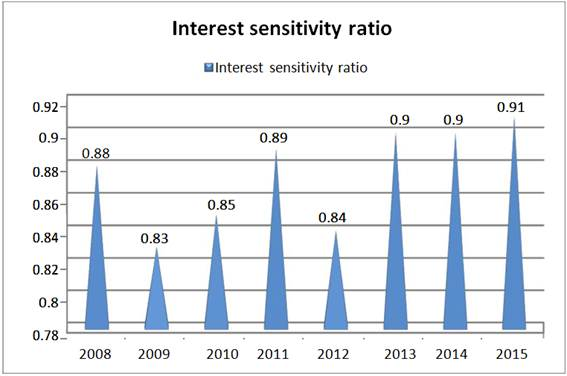Source: Agrani Bank´s annual report.

Figure 5. Interest Sensitivity Ratio.

4.3.2. Interest Rate Shock on Capital

Interest rate shock as an indicator of interest rate risks in the banking book and summarizes how it is used in the ongoing supervision of institutions in an environment of historically low interest rates and comparatively high market volatility. This indicator is calculated for standardized scenarios on the basis of institutions’ internal methods and procedures, and allows supervisors to observe the interest rate risks taken both by individual institutions and across all institutions.

Now I will show the effect of bank´s capital because of changing interest rate as mentioned below:

Table 7. Hypothetical Example of Interest Rate Shock on Capital.

 (BDT in million) Total Eligible 25240 Capital Total Risk 264690 weighted Assets Capital 9.54% Adequacy Ratio Assumed 1% 2% 3% 4% 5% Increase in Interest Rate Cumulative Gap -22493.18 -3974.81 14232.62 -101095.49 -47660.76 Earnings Impact -224.93 -79.50 426.98 -4043.82 -2383.04 on Cumulative Gap Capital after 25015.07 25160.50 25666.98 21196.18 22856.96 shock CAR after shock 9.45% 9.50% 9.7% 8% 8.63% Increase or -0.09% -0.05% 0.16% -1.54% -0.91% Decrease in CAR

Source: Agrani Bank´s annual report.

From above table, we can see that when Interest rate increased in the case of negative cumulative gap Capital Adequacy Ratio (CAR) also decreased. But in case of positive cumulative gap rises in interest rate also increase the Capital Adequacy Ratio. As UCBL has in position of negative cumulative gap rises in interest rate will loss the CAR. So, falling interest rate would be beneficial to the bank.

5. Comparative Discussion

Already I have shown two scheduled commercial banks´ individual condition in interest rate risk management. In there I have tried to show how they maintain it and after the period what is their condition. Now I will show a comparative overview between them. For this thing I choose some following facts.

1)  Net interest margin

2)  IS Gap

3)  Relative IS Gap

4)  Interest sensitivity ratio

By comparing this factor I will say which one is stayed in better condition. For this thing I have chosen a government commercial bank and a private commercial bank so that we can get an idea about the private and public commercial bank´s condition in maintaining interest rate risk management.

5.1. Position of Net Interest Margin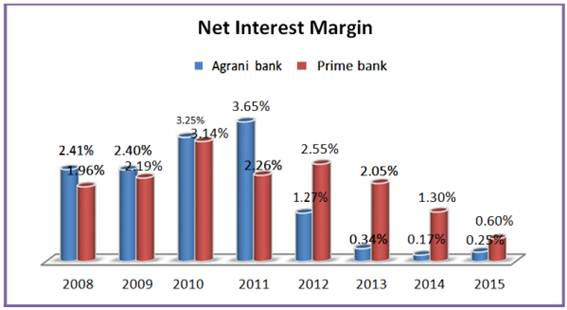Source: Annual report of Agrani and Prime Bank ltd.

Figure 6. Comparative position of Net Interest Margin.

Here we see that at the beginning Agrani bank NIM´s condition was much better then Prime bank but after 2011 they can´t able to hold this position for the next but in that time prime bank was in better position. Actually though the position of prime bank was not better then Agrani bank at beginning but their overall position was better than Agrani bank because their NIM was not so much fluctuated like Agrani bank.

5.2. Position of IS Gap

Table 8. IS Gap.

 (BDT in million) Year Agrani Bank Situation Prime bank Situation 2008 -22545.39 Liability Sensitive -3479 Liability Sensitive 2009 -34054.64 Liability Sensitive -3156 Liability Sensitive 2010 -36373.93 Liability Sensitive 143 Asset Sensitive 2011 -35863.28 Liability Sensitive -1319 Liability Sensitive 2012 -58476.42 Liability Sensitive -3842 Liability Sensitive 2013 -41029.51 Liability Sensitive -9440 Liability Sensitive 2014 -47532.91 Liability Sensitive -8930 Liability Sensitive 2015 -47660.97 Liability Sensitive -8771 Liability Sensitive

Source: Annual report of Agrani and Prime Bank ltd.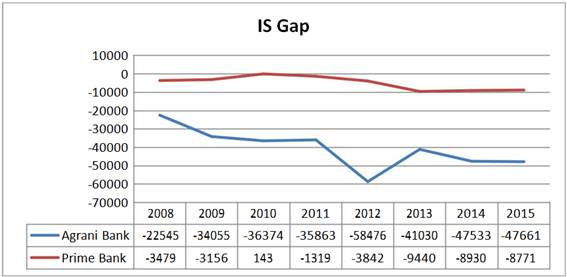Source: Annual report of Agrani and Prime Bank ltd.

Figure 7. Comparative IS GAP.

Here Prime bank is situated in very good condition rather than Agrani bank because their interest sensitive gap is huge and all are in liability sensitive condition so if interest rate raise then they will face a huge problem in that case Prime bank is situated in better condition though they also stay in liability sensitive position but their gap is not so much higher than Agrani bank and they also were in asset sensitive position in the year 2010.

5.3. Relative IS Gap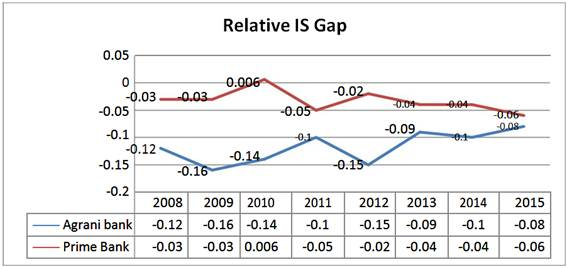Source: Annual report of Agrani and Prime Bank ltd.

Figure 8. Relative IS GAP Ratio.

Here prime bank´s condition is also much better than Agrani bank for all the year and in year 2010 their relative IS gap was also greater than zero which indicates in that year they have asset sensitive condition that means in interest rate fall down then they will face loss. Actually here main consideration fact is amount of IS gap if it is large then affect will be large and loss will be large and Agrani bank face this problem because their gap is so much larger than prime bank so if interest rate raise then they will be face huge loss but if interest rate fall down then will do huge gain so better is do maintain minimum gap so that not to face huge loss and according to this prime bank is situated in so much better position than Agrani bank.

5.4. Interest Sensitivity Ratio

Table 9. Interest Sensitivity Ratio.

 Year Agrani bank Prime bank 2008 0.88 0.97 2009 0.83 0.97 2010 0.85 1.00 2011 0.89 0.99 2012 0.84 0.98 2013 0.90 0.96 2014 0.90 0.96 2015 0.91 0.96

Source: Annual report of Agrani and Prime Bank ltd.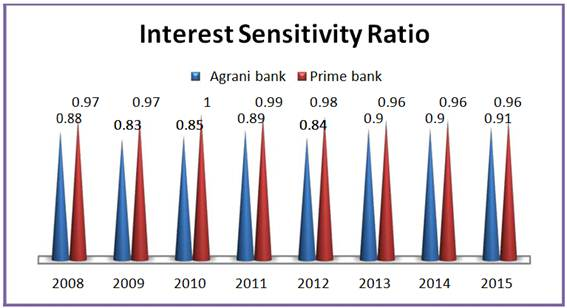Source: Annual report of Agrani and Prime Bank ltd.

Figure 9. Comparative Interest Sensitivity Ratio.

In this instance an Interest Sensitivity Ratio less than 1 tells us that we are looking at a liability sensitive institution. So we can say that Agrani bank Ltd is a liability sensitive institution and Prime bank limited is also situated in liability sensitive condition except in 2010. So we can say that here Prime bank is also situated in better position than Agrani bank.

5.5. Decision After Comparative Analysis

By considering all the things we can easily say that prime bank is stayed in better position in terms of interest rate risk management because they can able to maintain minimum gap between interest sensitive asset and interest sensitive liability and it is actually the main fact because here neither asset sensitive position nor liability sensitive position is good because we have no idea about the interest rate flow. It can be increased or can be decreased so best thing is maintain a minimum gap so that loss can´t be huge.

6. Findings

When completing the report I have found out several matters about Agrani Bank and Prime bank Ltd including the topic related information. As the topic is 'Interest Rate Risk Management', so I have found out the Bank's net interest margin conditions over the last 8 years, Interest Sensitive Gap position of the bank, Relative IS Gap of the bank, Interest sensitivity ratio of the bank & the effects of interest rates change in the profit of the bank. The following points will best describe the concept.

I. Agrani bank is not able to maintain minimum gap between interest sensitive asset and interest sensitive liability but Prime bank is able to maintain it. Their position is not the best but more satisfactory than Agrani bank.

II. Both the bank are stayed in liability sensitive position so it will be risky for them if interest rate rise but good thing for them is that now our interest rate is continuously falling down because of our slow economy so actually they are not staying in very danger position now but how many time it would be continued we have no idea. Actually they also don´t want it. They want a speedy economy and want higher interest rate in the economy.

III. The net interest margin is actually little satisfactory for ABL but it is fluctuated whichis not actually a good indication but for some years they abled to maintain it better than PBL.

IV. Banks actually can´t able to invest their deposited money properly they have huge deposit they can´t able to use it rapidly so a huge amount of idle money is stored in the bank which obviously increase the interest sensitive liability but can´t able to increase interest sensitive asset.

V. By analyzing eight years data of both banks it is clear that Agrani bank is stayed in more dangerous position than prime bank ltd.

VI. Both banks maintain large maturity liability than large maturity asset.

VII. According to sensitivity analysis Prime bank is better than Agrani bank.

VIII. Banks have calculated rate sensitive asset and liabilities within maturity bucket andapplied the sensitivity analysis to measure the level of Interest Rate shock on itscapital adequacy.

IX. Interest Rate Risk in the Banking Book of ABL and PBL, Capital adequacy ratiodecreases in case of negative cumulative gap and increases in case of positivecumulative gap.

7. Recommendations

Based on assessment of different aspects, the following recommendations have been made.

I. They have to increase interest sensitive asset especially Agrani bank.

II. Try to concentrate to asset management strategy that means try to more concentrate in loan than deposit because fund in available but can´t able to use it properly so need to give more priority in asset management strategy. Actually I think they can concentrate in relationship banking that means can maintain a individual for an individual client who always take information about him and give him advice if necessary if they can do it than they can do business only using some potential businessman.

III. Management must develop a correct interest rate forecast or find ways to reallocate earning asset and liabilities to increase the spread between interest revenue and interest expense.

IV. Banks can use IR derivate such as Future contract, Forward Contract, Interest Rate Swap and Options to reduce their IRR of the banks to the certain extent.

V. The effective risk management framework inclusive of Basel supervisory guidelines and BCBS principles can help the banks in a major base of controlling the loss exposed through IRR

VI. Bank must take conscious measure about capital adequacy ratio and abrupt changesin the interest rate.

VII. Bank can modify their duration gap of their asset and liability.

VIII. Management must choose the time period during which the net interest margin is tobe managed to achieve the desired value. Bank ensures that the interest rate risk is not included within the maturity risk.

IX. The central bank should play their role in standardization of interest spreads.

8. Conclusion

Interest is the main source of income for a bank. Measuring interest rate risk is a challenging task and is made even more difficult for depository institutions because of the uncertainty regarding core deposit behavior and the options embedded throughout their balance sheets. An adverse movement in interest rate risk may potentially: increase borrowing costs for borrowers, reduce returns for investors, reduce profitability of banks and reduce the net present value (NPV) of organizations due to the effect of changes in the discount rate (interest rate) on the value of financial instruments, hedges and the return on projects. So we can see that an adverse movement in the interest rate may cause loss for the bank if the bank fails to take proper steps for the management of interest rate risk. Interest Rate Risk Management is very important for any bank. As a state-owned bank, Agrani Bank Ltd is not performing well interest rate risk management but as a private commercial bank comparatively Prime bank can be able to perform very well in interest rate risk management which we can understand by seeing IS gap position of the bank and we can see that the bank is overall in a stable position in managing the interest rate risk. Though there is some irregularities have found but they successfully made over it. To prepare this thesis paper, I have tried to incorporate the necessary and relevant information in my report from my own personal experience in the bank as well as other relevant sources. The study was conducted by using the data of Agrani Bank and Prime bank Ltd from the year 2008 to 2015. At last I hope both bank can be able to overcome and able to reach at the top of the position in terms of interest rate risk management.

References

1. Rose, Peter. S. Commercial Bank Management. 5th ed. McGraw-Hill Higher Education.
2. Rose, P. and Hudgins, S. (2005). Bank Management & Financial Services. 6th ed. McGraw-Hill Higher Education, pp.484-503.
3. AmadouSy, IMF Working Paper on Managing the Interest Rate Risk of Indian Banks’overnment Securities Holdings.
4. Antonios, A., Huainan, Z., and Bilei, Z. (2009), Corporate debt issues and interest rate risk management: Hedging or market timing? Journal of Financial Markets.
5. Basel Committee on Banking Supervision, Bank for International Settlements Press & Communications, CH-4002 Basel, Switzerland.
6. BIS(Bank for Interational settlement), 1997, "Principles for the Management and Supervision of Interest Rate Risk," Basel Committee on Banking Supervision.
7. BIS(Bank for Interational settlement), 2003, "Principles for the Management and Supervision of Interest Rate Risk," Basel Committee on Banking Supervision.
8. Bartram, S. (2002), The Interest Rate Exposure of Nonfinancial Corporations. European Finance Review.
9. Bessler, W. & Booth, G. G. (1994), ‘An interest rate risk management model for commercial banks’, European Journal of Operational Research.
10. Boukrami, L. (2003), The Use of Interest Rate Swaps by Commercial Banks.
11. (Electronic) Available: Social Science Research Network. (2003-12-18).
12. CAYMAN ISLANDS MONETARY AUTHORITY- 'Statement of Guidance on Interest Rate Risk Management'
13. De Nederlandsche Bank N.V. Guidelines on interest rate risk in the banking book, July 2005.
14. Heffernan, S., (1996), Modern Banking in theory and practice. New York: John Wiley and Sons.

 Contents 1. 2. 3. 3.1. 3.2. 3.3. 4. 4.1. 4.2. 4.3. 5. 5.1. 5.2. 5.3. 5.4. 5.5. 6. 7. 8.
Article ToolsAbstractPDF(435K)